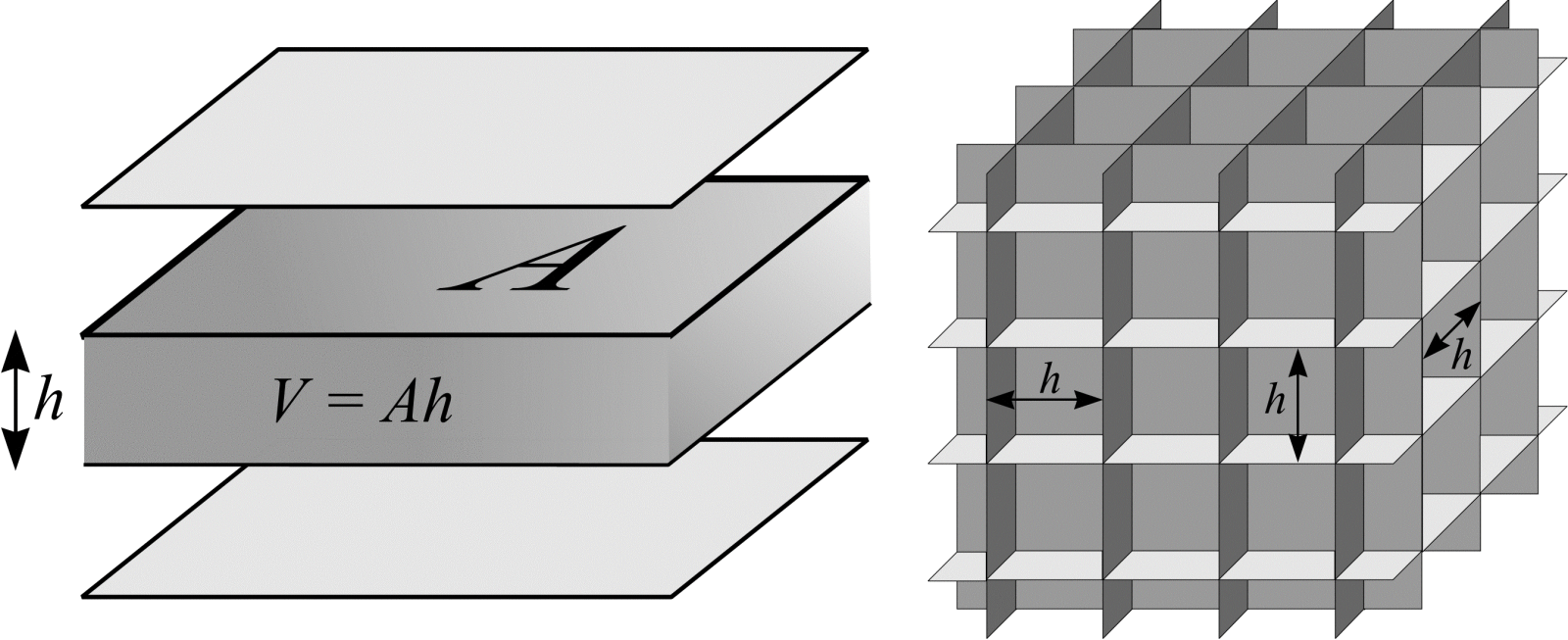# Curve length in $$\real^{3}$$

In a previous section it is shown how Buffon's needle problem in two dimensions can be readily extended to facilitate calculation of curve length in $$\real^{2}$$. In exactly the same way, Buffon's needle in three dimensions can be extended to estimate the length, $$L$$, of a curve, $$C$$, in $$\real^{3}$$. The curve $$C$$ is thrown isotropic uniform randomly into the array of planes.

Suppose the curve $$C$$ is approximated by a union of line segments, $$Y = \bigcup(y_{i})_{i=1}^{n} = \{y_{1}, y_{2}, ..., y_{i}, ..., y_{n}\}$$. If the length of $$y_{i}$$ is $$l_{i}$$ $$(1 \le i \le n)$$, an approximation of the curve length of $$C$$ is $$L = \sum_{i=1}^{n} l_{i}$$. By comparison with (38), the probability that the line segment $$y_{i}$$ intersects a joint is $$l_{i}/2h$$. Furthermore, (39) implies the length of $$y_{i}$$ is $$l_{i} = 2 h \mathbf{E}Q_{i}$$. $$Q_{i}$$ is a discrete random variable that maps the outcomes $$y_{i}$$ transects a plane and $$y_{i}$$ falls between planes to the real numbers 1 and 0, respectively. Let the total number of transects, $$Q$$, between the planes and $$Y$$ be denoted as the sum $$Q_{1} + Q_{2} + ... + Q_{n}$$. Taking into account all line segments:

\begin{align} L &= 2 \cdot h \cdot \sum_{i=1}^{n} \mathbf{E}Q_{i} \\ &= 2 \cdot h \cdot \sum_{i=1}^{n} \mathbf{E}Q. \end{align} \tag{41}

An estimate of $$L$$ can be obtained from a single throw of $$Y$$. An estimate of $$L$$ is

\begin{align} \hat{L} &= 2 \cdot h \cdot \sum_{i=1}^{n} Q_{i} \\ &= 2 \cdot h \cdot Q. \end{align} \tag{42}

Finally, consider again the systematic set of parallel planes a distance $$h$$ apart. The average area of test plane likely to fall within a certain volume can be calculated. Figure 15(a) shows an area of test plane, $$A$$, and the volume it occupies. The test system of planes has area per unit volume $$A/V = A/Ah = 1/h$$. Next, substitute $$h = V/A$$ into (41). The result is an important formula originally due to Saltykov (1946):

\begin{align} \frac{L}{V} &= 2 \cdot \frac{\mathbf{E}Q}{A} \\ \text{and so } L &= 2 \cdot \frac{V}{A} \cdot \mathbf{E}Q. \end{align} \tag{43}

An estimate of $$L$$ can be obtained from a single throw of $$Y$$:

$$\hat{L} = 2 \cdot \frac{V}{A} \cdot Q. \tag{44}$$

Suppose a needle, length $$L$$ $$(L > h)$$, is thrown with IUR position onto a test system of parallel planes. $$\hat{L}$$ is greatest when the needle is perpendicular to the test planes and smallest when the needle is parallel to the test planes. A better estimation of $$L$$ is obtained if the test system of parallel planes is replaced by a test system of three sets of mutually orthogonal planes (see Figure 15(b)). Such a test system has three times as much area per unit volume as the parallel plane test system so that $$A/V = 3 \cdot (1/h)$$.(a)
(b)

Figure 15: Test systems of (a) parallel planes ($$A/V = 1/h$$) and (b) mutually orthogonal planes ($$A/V = 3/h$$). The figure shows each test system with the volume, $$V$$, an area of test surface, A, occupies.

## References

SALTYKOV, S. A. The method of intersections in metallography (In Russian). Zavodskaja laboratorija, 12, 816-825 (1946).